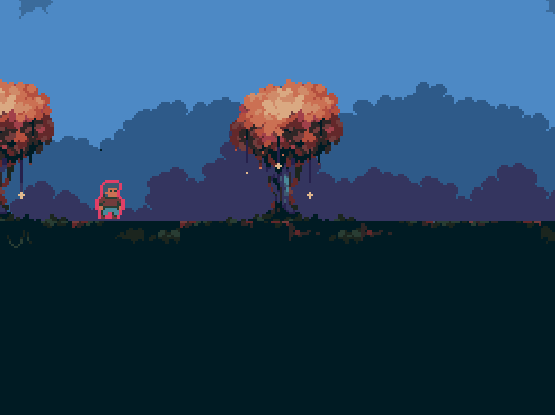Instantly share code, notes, and snippets.

# superqix/pixeloutline.lua

Last active March 10, 2023 17:30
Pixel prefect outline shader in Solar2D
This file contains bidirectional Unicode text that may be interpreted or compiled differently than what appears below. To review, open the file in an editor that reveals hidden Unicode characters. Learn more about bidirectional Unicode characters
 --local object = display.newImage("image.png") --object.fill.effect = "filter.custom.pixeloutline" --object.fill.effect.intensity = 0.0 to 10.0 -- how thick is it --object.fill.effect.r, g, b = 4 to 50 -- color local kernel = {} kernel.language = "glsl" kernel.category = "filter" kernel.name = "pixeloutline" kernel.vertexData = { { name = "r", default = 0, min = 0, max = 1, index = 0, },{ name = "g", default = 0, min = 0, max = 1, index = 1, },{ name = "b", default = 0, min = 0, max = 1, index = 2, },{ name = "size", default = 1, min = 0, max = 4, index = 3, }, } kernel.fragment = [[ P_NORMAL float size = CoronaVertexUserData.w; P_COLOR vec4 FragmentKernel( P_UV vec2 texCoord ) { P_COLOR vec4 texColor = texture2D( CoronaSampler0, texCoord ); if (texColor.a == 0.0) { P_NORMAL float w = size * CoronaTexelSize.x; P_NORMAL float h = size * CoronaTexelSize.y; if (texture2D(CoronaSampler0, texCoord + vec2(w, 0.0)).a != 0.0 || texture2D(CoronaSampler0, texCoord + vec2(-w, 0.0)).a != 0.0 || texture2D(CoronaSampler0, texCoord + vec2(0.0, h)).a != 0.0 || texture2D(CoronaSampler0, texCoord + vec2(0.0,-h)).a != 0.0) texColor.rgba = vec4(CoronaVertexUserData.x,CoronaVertexUserData.y,CoronaVertexUserData.z,1); } return CoronaColorScale(texColor); } ]] graphics.defineEffect( kernel )

### superqix commented Nov 18, 2021 • edited

So you will need to leave a 1px border around the image for the outline to work. In this example there's no border on the bottom, so his feet aren't outlined.